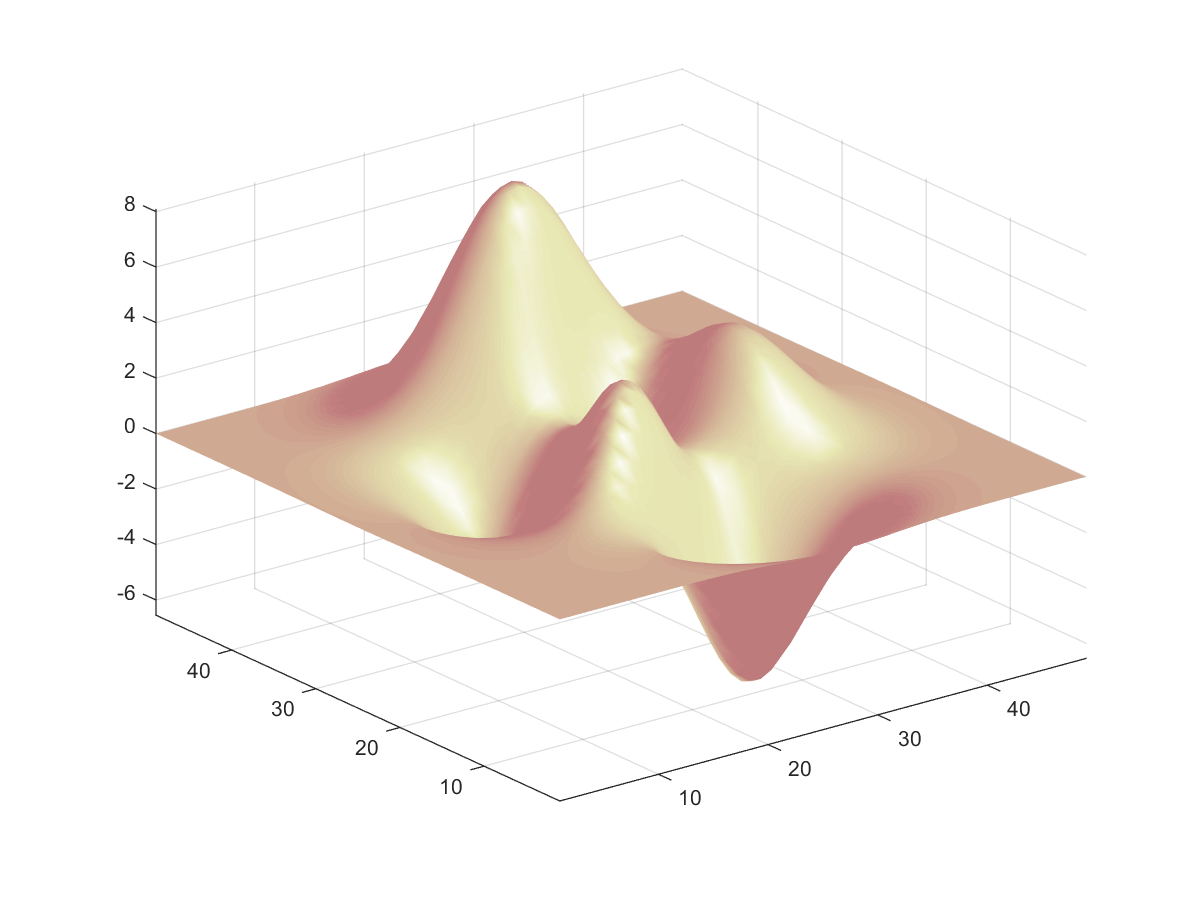4-3 嚙踝蕭嚙踝蕭嚙踝蕭嚙踝蕭嚙踝蕭嚙踝蕭嚙踝蕭嚙踝蕭嚙踝蕭嚙踝蕭嚙?

Example 1: 04-三維立體繪圖/colorbar01.mpeaks; colorbar; z = 3*(1-x).^2.*exp(-(x.^2) - (y+1).^2) ... - 10*(x/5 - x.^3 - y.^5).*exp(-x.^2-y.^2) ... - 1/3*exp(-(x+1).^2 - y.^2)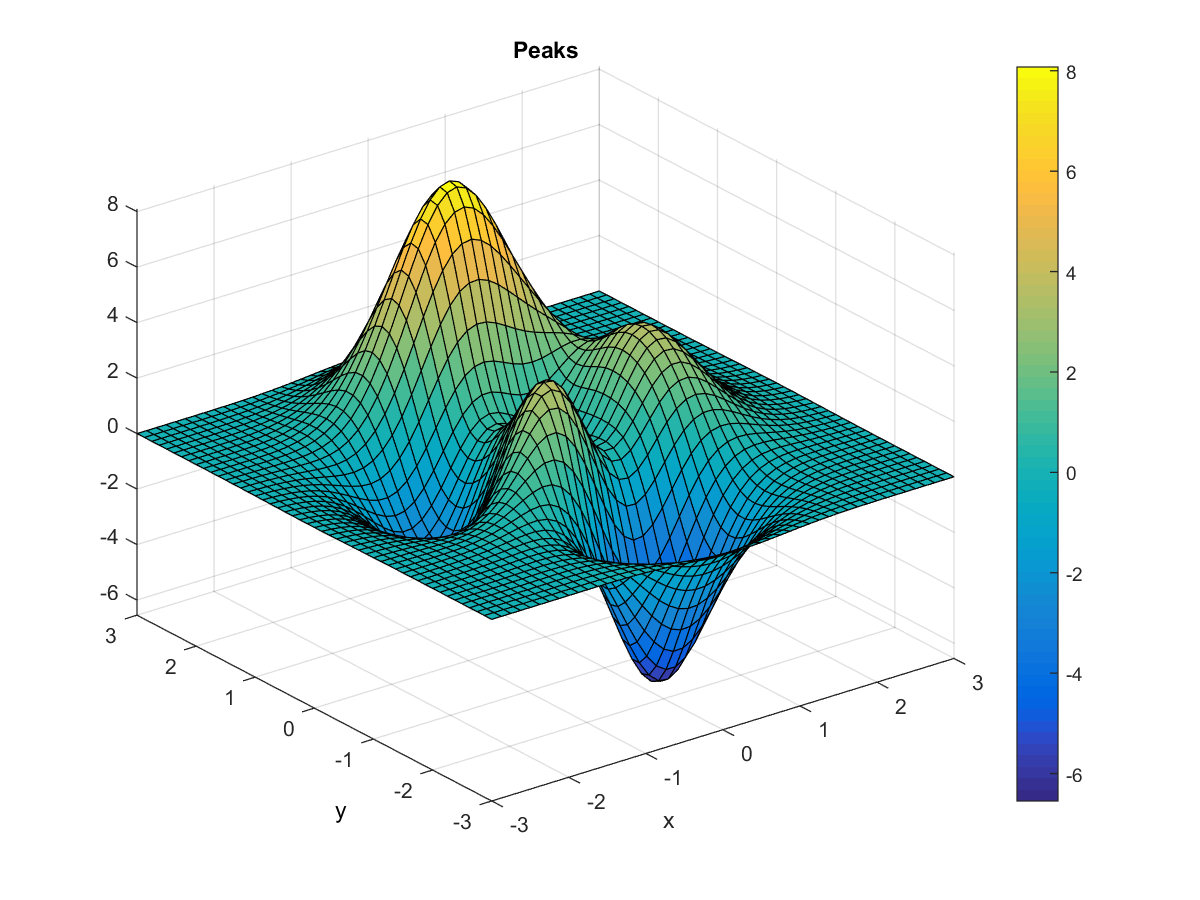black（黑） 0 0 0
white（白） 1 1 1
red（紅） 1 0 0
green（綠） 0 1 0
blue（藍） 0 0 1
yellow（黃） 1 1 0
magenta（錳紫） 1 0 1
cyan（青藍） 0 1 1
gray（灰） 0.5 0.5 0.5
dark red（暗紅） 0.5 0 0
copper（銅色） 1 0.62 0.4
aquamarine（碧綠） 0.49 1 0.83

MATLAB 預設的顏色對應表可由 colormap 得知，如下：

Example 2: 04-三維立體繪圖/colormap01.mcm=colormap; size(cm) ans = 64 3

Example 3: 04-三維立體繪圖/plotxyz13.mpeaks; colormap(rand(64,3)); colorbar; z = 3*(1-x).^2.*exp(-(x.^2) - (y+1).^2) ... - 10*(x/5 - x.^3 - y.^5).*exp(-x.^2-y.^2) ... - 1/3*exp(-(x+1).^2 - y.^2)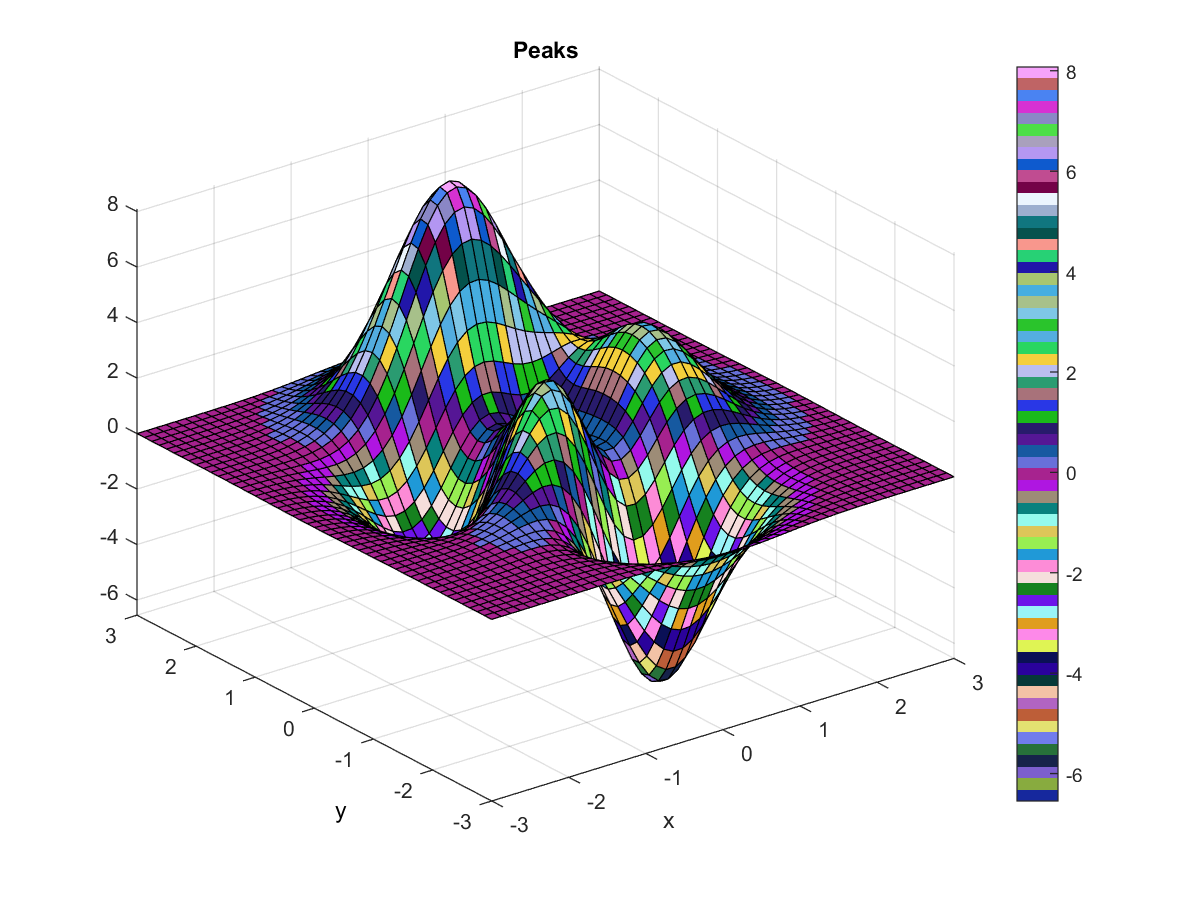colormap hsv HSV 的顏色對應表（預設值）
colormap hot 代表“熱”的顏色對應表
colormap cool 代表“冷”的顏色對應表
colormap summer 代表“夏天”的顏色對應表
colormap gray 代表“灰階”的顏色對應表
colormap copper 代表“銅色”的顏色對應表
colormap autumn 代表“秋天”的顏色對應表
colormap winter 代表“冬天”的顏色對應表
colormap spring 代表“春天”的顏色對應表
colormap bone 代表“X光片”的顏色對應表
colormap pink 代表“粉紅”的顏色對應表
colormap flag 代表“旗幟”的顏色對應表

Example 4: 04-三維立體繪圖/plotxyz14.mpeaks; colormap cool; colorbar z = 3*(1-x).^2.*exp(-(x.^2) - (y+1).^2) ... - 10*(x/5 - x.^3 - y.^5).*exp(-x.^2-y.^2) ... - 1/3*exp(-(x+1).^2 - y.^2)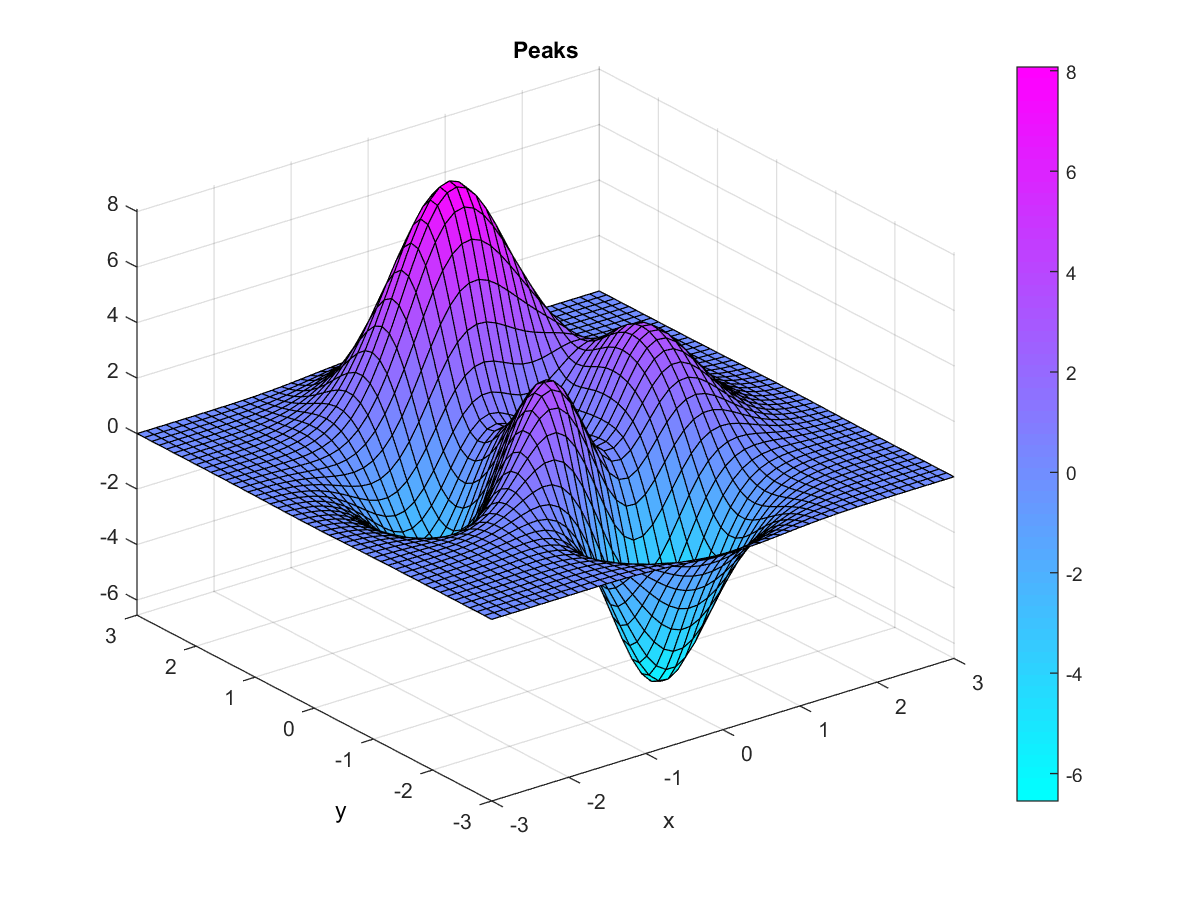Example 5: 04-三維立體繪圖/plotxyz15.m[X, Y, Z] = peaks; surf(X, Y, Z, gradient(Z)); axis tight; colormap hot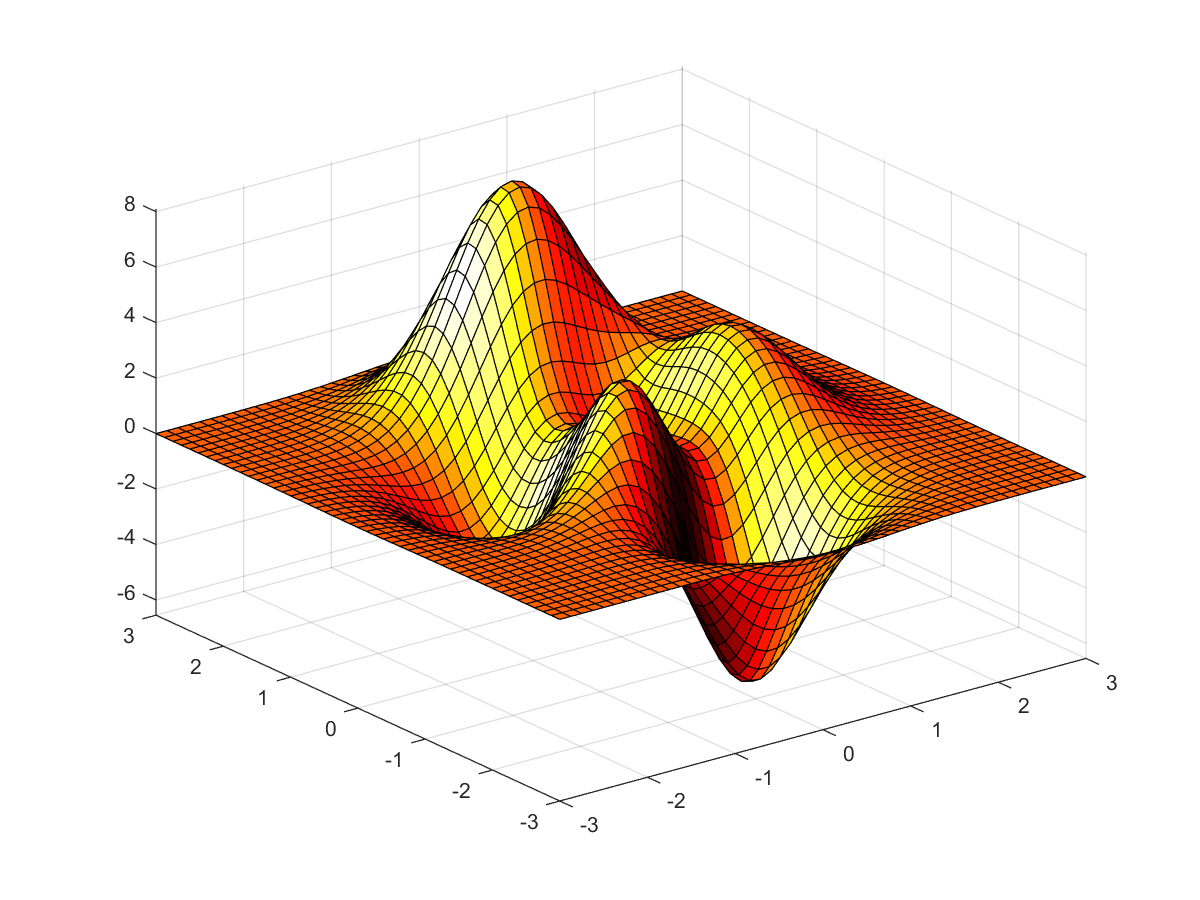Example 6: 04-三維立體繪圖/plotxyz16.m[X, Y, Z] = peaks; surf(X, Y, Z, del2(Z)); axis tight; colormap hot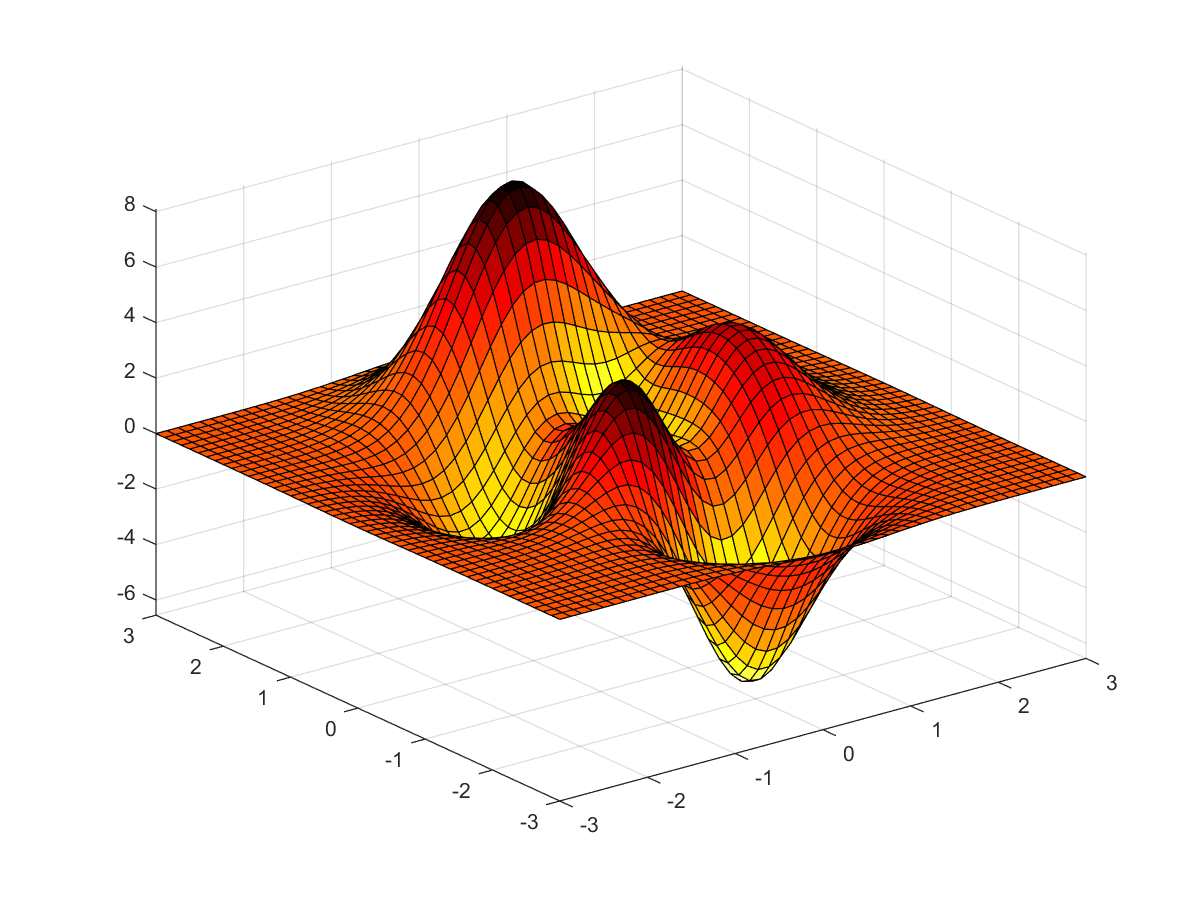Example 7: 04-三維立體繪圖/plotxyz17.mcolormap copper subplot(3, 1, 1); rgbplot(colormap); brighten(colormap, 0.5) subplot(3, 1, 2); rgbplot(colormap); brighten(colormap, -0.8) subplot(3, 1, 3); rgbplot(colormap);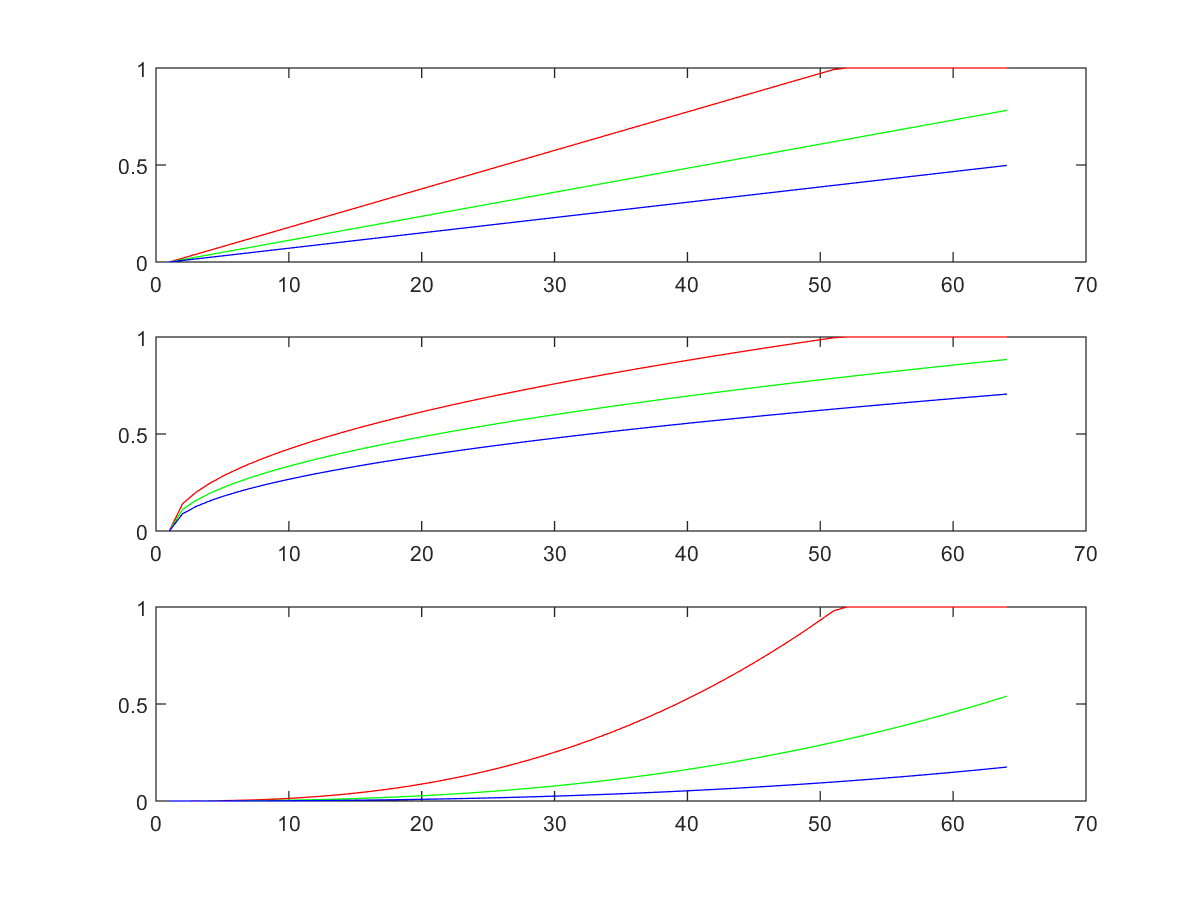Example 8: 04-三維立體繪圖/plotxyz18.mZ = peaks(50); C(:, :, 1) = rand(50); % C(:,:,1) 代表 R（Red，紅色）的份量 C(:, :, 2) = rand(50); % C(:,:,2) 代表 G（Green，綠色）的份量 C(:, :, 3) = rand(50); % C(:,:,3) 代表 B（Blue，藍色）的份量 surf(Z, C); axis tight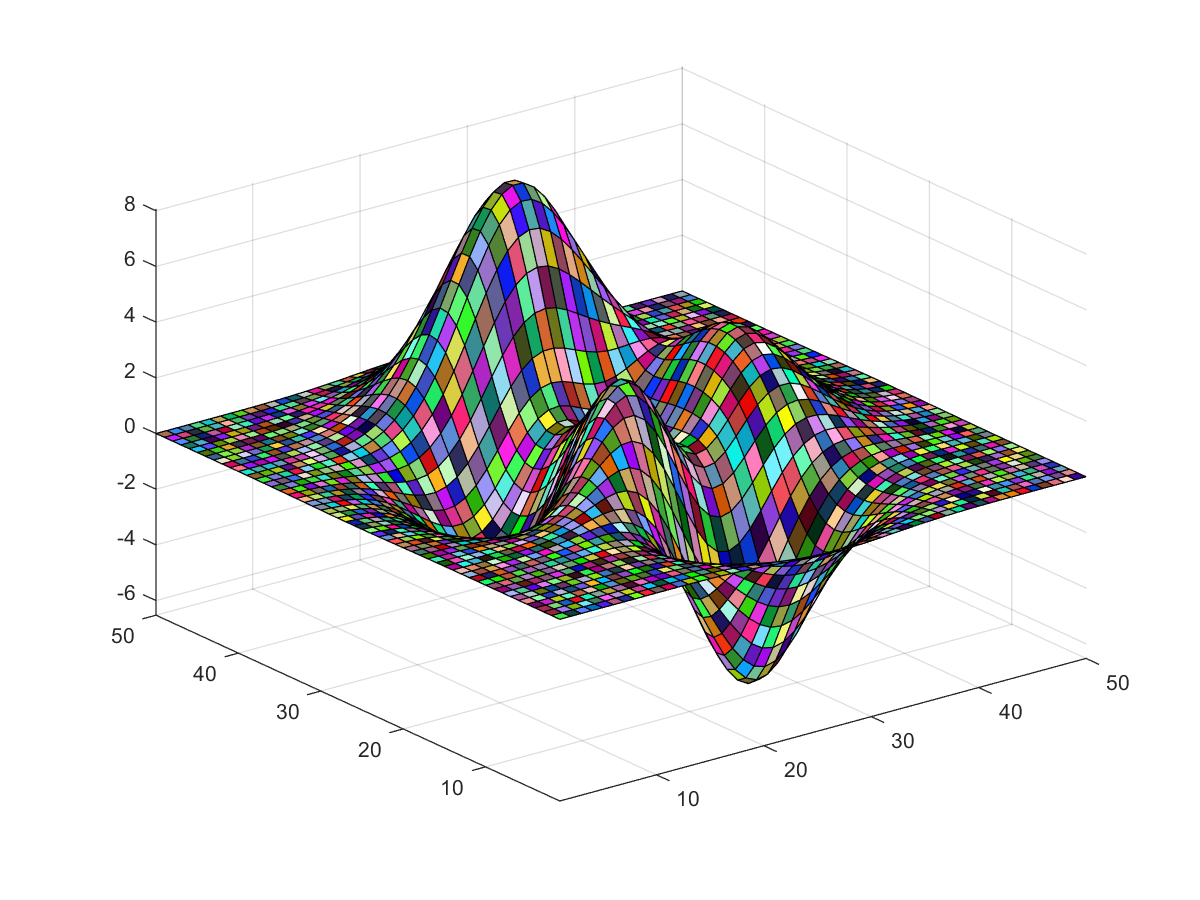Example 9: 04-三維立體繪圖/shadingInterp01.mpeaks; colormap jet; colorbar; shading interp; z = 3*(1-x).^2.*exp(-(x.^2) - (y+1).^2) ... - 10*(x/5 - x.^3 - y.^5).*exp(-x.^2-y.^2) ... - 1/3*exp(-(x+1).^2 - y.^2)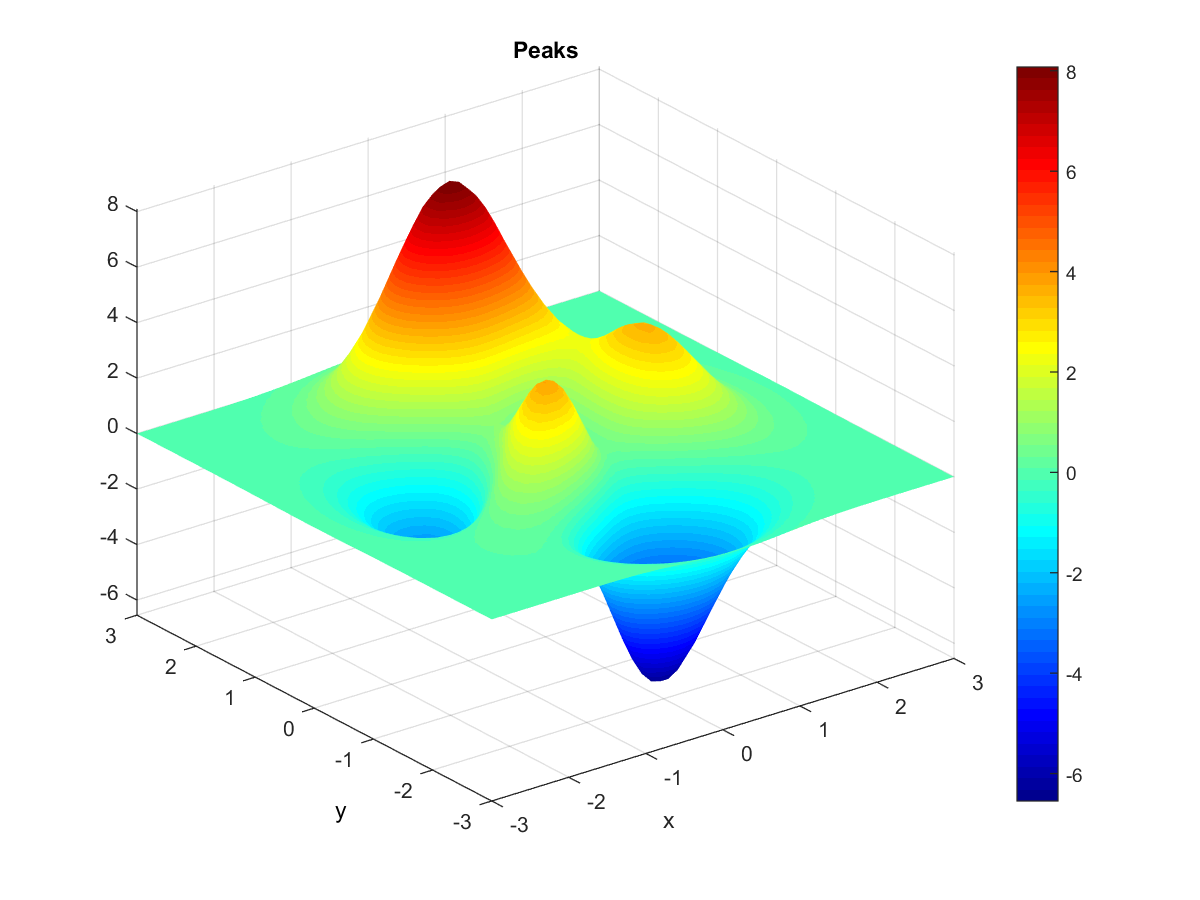shading interp 使用 Bilinear Interpolation 來使「瓷磚」根據四頂點的顏色產生連續的變化